C.I. Calculator: Single Incidence Rate

Data Input: (Help) (Example)

 Input Result 1-α Rate a Lower N Upper

Note:

 Variables Descriptions 1-α Two-sided confidence level a Number of clinical events N Person-time at risk Rate Incidence rate Lower Lower C.I. Upper Upper C.I.

Help Aids Top

Description:

Incidence rate is the rate at which new clinical events occur in a population.

It is the number of new events divided by the population at risk of an event in a specific time period, sometimes it is the person-time at risk.

Procedure:

1. Enter

a)    Value of 1-α, the two-sided confidence level

b)    Value of a, the number of clinical events

c)    Value of N, the person-time at risk

1. Click the button “Calculate” to obtain the lower and upper endpoints of 100(1-α)% confidence interval
1. Click the button “Reset” for another new calculation

Formula:

Define: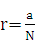Define the standard error: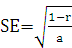The 100(1-α)% confidence interval is defined as: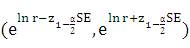Notation:

100(1-α)% confidence interval: We are 100(1-α)% sure the true value of the parameter is included in the confidence interval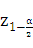: The z-value for standard normal distribution with left-tail probability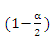Example

Suppose the number of new cases is 9 (a = 9)

Person-year at risk is 4028.16 (N = 4028.16)

Then the incidence rate is 0.0022343 and

The 95% confidence interval ((1-α) =0.95) is (0.0011634, 0.004291)

Top